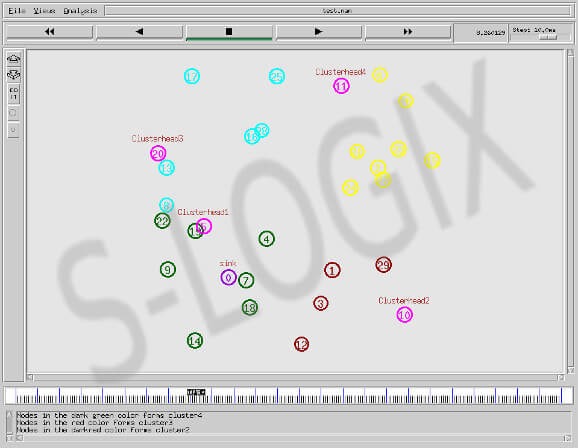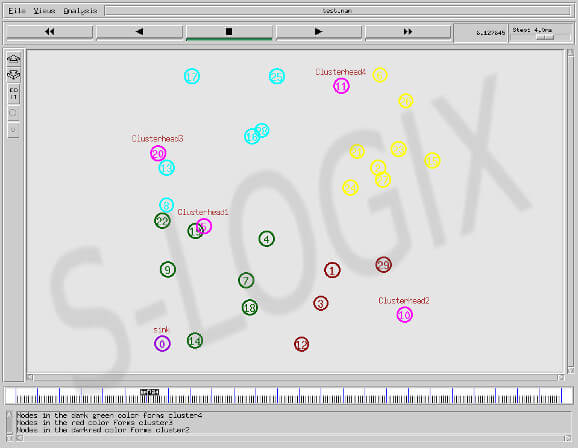• #5, First Floor, 4th Street Dr. Subbarayan Nagar Kodambakkam, Chennai-600 024 Landmark : Samiyar Madam
• pro@slogix.in
• +91- 81240 01111

How to create the shortest path for mobile sink in NS2?

Description

In the sensor network after clusterhead selection, cluster member sends data to clusterhead. Clusterhead aggregates the received data from cluster member. In mobile sink network, only the sink moves to collect data from clusterhead whereas in static sink case, data is sent in multiple hops to sink from clusterhead. So sink mobility scheme achieves energy optimization with certain delay due to the time taken for the arrival sink nearer to clusterhead. Hence the sink moves along the path as per the shortest path algorithm. The code given below provides the logic for shortest path in grid based clustering.

Sample Code

Initialize the variable
mc=0;
ec =1;
mx(mc), my(mc) = list of coordinates to which the mobile sink is required to move
for ( j= 1;j <= number_grids; j++) {
mpn = ec%2;
if ( mpn == 1) {
for ( i= 1;i <= number_grids; i++) { 　　　　 px = (i-1 +0.5)*(communication_range); 　　　　py = (j-1 +0.5)*(communication_range) 　　　　 mx(mc) =px; 　　　　 my(mc) =py 　　　　 mc =mc+1; 　　　　} 　　　　} else { 　　　　for (i= number_grids; i >= 1; i-1 ) {
px = (i-1 +0.5)*(communication_range);
py = (j-1 +0.5)*(communication_range)
mx(mc) =px;
my(mc) =py
mc =mc+1;
}
}
ec = ec +1;
}

Screenshots

Sink node starting position is (0, 0).

After some time, sink node moves to collect data from clusterhead 6.